# Acceleration + motion problems - math problems

#### Number of problems found: 29

• At what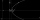At what speed did the motorist drive when he reacted by noticing the obstacle by 0.8 s; the magnitude of the opposite acceleration during braking was 6.5 m/s ^ 2 and the car ran to a stop track 35 m?
• The satellite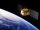The satellite orbiting the Earth at an altitude of 800 km has a speed of 7.46 km/s. For how long would it have to move from the start to the orbit to reach this speed if it evenly accelerated its motion in a straight line? What is the acceleration of sate
• The train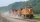The train starts for 2 min with an acceleration of 25 cm/s2. What speed will he gain in this time and what path will he pass?
• The cyclist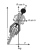The cyclist reaches a speed of 32 km/h in 3.4 seconds. What is its acceleration in m/s2?
• The projectile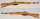The projectile leaves the 10 m long barrel at an instantaneous speed of 500 m/s. What is the acceleration of the movement of the projectile in the barrel and in what time will the projectile pass the barrel if, we assume that the movement was evenly accel
• The bomber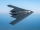From what distance in front of the target must a parachute load be dropped from an aircraft flying at an altitude of 1260 m if it slopes at a speed of 5.6 m/s and at the same time is carried in the direction of movement at a speed of 12 m/s. What is the d
• Two cars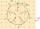Two cars, spaced d1 = 500 m apart, start moving against each other at time t1 = 0, the first car with acceleration a1 = 4 m/s ^ 2 and the second with acceleration a2 = 6 m/s ^ 2. At what time t2 do they meet? What distances s1 and s2 will they move from t
• Free fall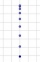For how long and at what speed does the body fall to the ground during a free fall from a height of 35 m?
• Up and down motionWe throw the body from a height h = 5 m above the Earth vertically upwards v0 = 10 m/s. How long before we have to let the second body fall freely from the same height to hit the Earth at the same time?
• Braking distance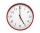The car travels at an average speed of 12 km/h and detects an obstacle 10 m in front of it. At 1 m in front of the obstacle it already runs 2 km/h. What is the braking distance? What is the required deceleration for stop in: A) 1m B) 1s?
• GravitationFrom the top of the 80m high tower, the body is thrown horizontally with an initial speed of 15 m/s. At what time and at what distance from the foot of the tower does the body hit the horizontal surface of the Earth? (use g = 10 ms-2)
• Rocket start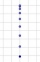The body launched vertically up returns to the start site in 6 seconds. What height did it have?
• A carA car weighing 1.05 tonnes driving at the maximum allowed speed in the village (50 km/h) hit a solid concrete bulkhead. Calculate height would have to fall on the concrete surface to make the impact intensity the same as in the first case!
• Free fallThe free fall body has gone 10m in the last 0.5s. Find the body speed at the moment of impact.
• DriverThe car driver drives at a speed of 100 km/h, saw the obstacle, and began to brake with a slowing of 5 m/s². What is the length of the pathway to stopping the car when the driver has registered the obstacle with a delay of 0.7 s?
• Brakes of a carFor the brakes of a passenger car to be effective, it is prescribed that a car be moving on a horizontal road at a speed of 40 km. A car must stop on the track 15.4 m. What is the deceleration of the car?
• Car overtaking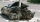A passenger car travels at a speed of 30 m/s, and before it travels freight truck that drives at a constant speed of 10 m/s. Within 30 meters of the truck, the driver of the car finds out that the truck can not overtake. That's why it starts braking with
• An accelerationThe car goes on a straight road at a speed of 72 km/h. At some point, the driver starts to brake and stops the car in 5 seconds. Find: (a) the acceleration during braking (b) the distance traveled during braking.
• BrakesThe braking efficiency of a passenger car is required to stop at 12.5 m at an initial speed of 40 km/h. What is the acceleration braking by brakes?
• Train speed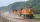The train speed is decreased during 50 sec from 72 km/h to 36 km/h. Assuming that the train movement is equally slowing, find the the acceleration and the distance that it travels at.

Do you have an exciting math question or word problem that you can't solve? Ask a question or post a math problem, and we can try to solve it.

We will send a solution to your e-mail address. Solved examples are also published here. Please enter the e-mail correctly and check whether you don't have a full mailbox.

Acceleration - math problems. Motion problems - math problems.